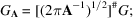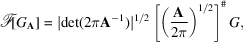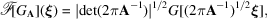International
Tables for
Crystallography
Volume B
Reciprocal space
Edited by U. Shmueli

International Tables for Crystallography (2010). Vol. B, ch. 1.3, pp. 38-39   | 1 | 2 |

## Section 1.3.2.4.4.2. Gaussian functions and Hermite functions

G. Bricognea

aGlobal Phasing Ltd, Sheraton House, Suites 14–16, Castle Park, Cambridge CB3 0AX, England, and LURE, Bâtiment 209D, Université Paris-Sud, 91405 Orsay, France

#### 1.3.2.4.4.2. Gaussian functions and Hermite functions

| top | pdf |

Gaussian functions are particularly important elements of. In dimension 1, a well known contour integration (Schwartz, 1965, p. 184) yieldswhich shows that the `standard Gaussian'is invariant underand. By a tensor product construction, it follows that the same is true of the standard Gaussianin dimension n:In other words, G is an eigenfunction ofandfor eigenvalue 1 (Section 1.3.2.4.3.4).

A complete system of eigenfunctions may be constructed as follows. In dimension 1, consider the family of functionswhere D denotes the differentiation operator. The first two members of the familyare such that, as shown above, andhenceWe may thus take as an induction hypothesis thatThe identitymay be writtenand the two differentiation theorems give:Combination of this with the induction hypothesis yieldsthus proving thatis an eigenfunction offor eigenvaluefor all. The same proof holds for, with eigenvalue. If these eigenfunctions are normalized asthen it can be shown that the collection of Hermite functionsconstitutes an orthonormal basis ofsuch thatis an eigenfunction of(respectively) for eigenvalue(respectively).

In dimension n, the same construction can be extended by tensor product to yield the multivariate Hermite functions(whereis a multi-index). These constitute an orthonormal basis of, withan eigenfunction of(respectively) for eigenvalue(respectively). Thus the subspacesof Section 1.3.2.4.3.4are spanned by thosewith.

General multivariate Gaussians are usually encountered in the nonstandard formwhere A is a symmetric positive-definite matrix. Diagonalizing A aswiththe identity matrix, and putting, we may writei.e.hence (by Section 1.3.2.4.2.3)i.e.i.e. finallyThis result is widely used in crystallography, e.g. to calculate form factors for anisotropic atoms (Section 1.3.4.2.2.6) and to obtain transforms of derivatives of Gaussian atomic densities (Section 1.3.4.4.7.10).

### References

Schwartz, L. (1965). Mathematics for the Physical Sciences. Paris: Hermann, and Reading: Addison-Wesley.